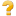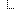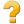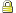﻿ Extension: calculatedValue - FHIR v4.5.0

# Extension: calculatedValue

 FHIR Infrastructure Work Group Maturity Level: 1 Informative Use Context: Any

URL for this extension:

`http://hl7.org/fhir/StructureDefinition/cqf-calculatedValue`

Status: draft. Extension maintained by: Health Level Seven, Inc. - CDS WG

The name of an expression in a referenced library that determines a calculated value.

Context of Use: Use on Element ID Element

## Extension Content

Summary

NameFlagsCard.TypeDescription & ConstraintscalculatedValue0..*stringURL = http://hl7.org/fhir/StructureDefinition/cqf-calculatedValue
calculatedValue: The name of an expression in a referenced library that determines a calculated value.

Use on Element ID ElementDocumentation for this format

Full Structure

NameFlagsCard.TypeDescription & Constraintsextension0..*ExtensionURL = http://hl7.org/fhir/StructureDefinition/cqf-calculatedValue
calculatedValue: The name of an expression in a referenced library that determines a calculated value.

Use on Element ID Elementextension I0..0url 1..1uri"http://hl7.org/fhir/StructureDefinition/cqf-calculatedValue"value[x] I1..1stringValue of extensionDocumentation for this format

XML Template

```<!-- calculatedValue --><extension xmlns="http://hl7.org/fhir"
url="http://hl7.org/fhir/StructureDefinition/cqf-calculatedValue" >
<!-- from Element: extension -->
<valueString value="[string]"/><!--1..1 Value of extension -->
</extension>
```

JSON Template

```{ // calculatedValue
// from Element: extension
"extension" : [ //  sliced by value:url  in the specified order, Open ]
"url" : "http://hl7.org/fhir/StructureDefinition/cqf-calculatedValue", // R!
"valueString" : "<string>" // C? R! Value of extension
}
```

Summary

NameFlagsCard.TypeDescription & ConstraintscalculatedValue0..*stringURL = http://hl7.org/fhir/StructureDefinition/cqf-calculatedValue
calculatedValue: The name of an expression in a referenced library that determines a calculated value.

Use on Element ID ElementDocumentation for this format

Full Structure

NameFlagsCard.TypeDescription & Constraintsextension0..*ExtensionURL = http://hl7.org/fhir/StructureDefinition/cqf-calculatedValue
calculatedValue: The name of an expression in a referenced library that determines a calculated value.

Use on Element ID Elementextension I0..0url 1..1uri"http://hl7.org/fhir/StructureDefinition/cqf-calculatedValue"value[x] I1..1stringValue of extensionDocumentation for this format

XML Template

```<!-- calculatedValue --><extension xmlns="http://hl7.org/fhir"
url="http://hl7.org/fhir/StructureDefinition/cqf-calculatedValue" >
<!-- from Element: extension -->
<valueString value="[string]"/><!--1..1 Value of extension -->
</extension>
```

JSON Template

```{ // calculatedValue
// from Element: extension
"extension" : [ //  sliced by value:url  in the specified order, Open ]
"url" : "http://hl7.org/fhir/StructureDefinition/cqf-calculatedValue", // R!
"valueString" : "<string>" // C? R! Value of extension
}
```

Constraints

• ele-1: All FHIR elements must have a @value or children (xpath: @value|f:*|h:div)
• ext-1: Must have either extensions or value[x], not both (xpath: exists(f:extension)!=exists(f:*[starts-with(local-name(.), 'value')]))
• ele-1: On Extension.extension: All FHIR elements must have a @value or children (xpath on Extension.extension: @value|f:*|h:div)
• ext-1: On Extension.extension: Must have either extensions or value[x], not both (xpath on Extension.extension: exists(f:extension)!=exists(f:*[starts-with(local-name(.), "value")]))
• ele-1: On Extension.value[x]: All FHIR elements must have a @value or children (xpath on Extension.value[x]: @value|f:*|h:div)